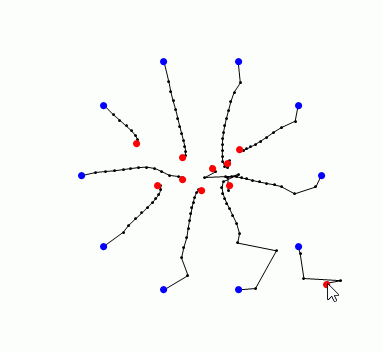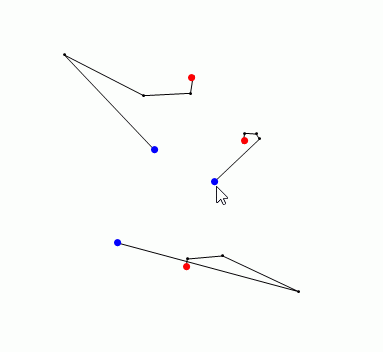Conversation

Professor: We’re going to talk about Taylor polynomials this quarter.
Student: Shit.
Professor: What was that? I thought I heard yippee!

If you ever take the inverse of a matrix and I…

If you ever take the inverse of a matrix and I find out, I will kill you.

If you ever take the inverse of a matrix and I…

If you ever take the inverse of a matrix and I find out, I will kill you.

If you all make me really moody, I will give y…

If you all make me really moody, I will give you a 5×5 determinant to do by hand for the final – that’s 120 terms.

We cannot analyze ideas. We only analyze algor…

We cannot analyze ideas. We only analyze algorithms here.

“If you’ve seen The Matrix, you’ll understand binary.”

“If you’ve seen The Matrix, you’ll understand binary.”

Numerical analysis professor

hyrodium: Durand-Kerner method is a root-finding algorithm for…Durand-Kerner method is a root-finding algorithm for solving polynomial equations.

The red points are the solution of P(z)=0, and the blue points is a initial values z_k^(0).

Here’s mathematica code, and you can try this code on Wolfram Programming Lab for free! (https://www.wolfram.com/programming-lab/):

m = 20;
pts2[pts_,
n_] := {(Complex @@@ pts)[[1 ;; n]], (Complex @@@ pts)[[-n ;; -1]]};
CompList[lz_] := Transpose@{Re[#], Im[#]} &@lz;
DKA[root_, lz0_] := (
Clear[f, rec, lz];
lz = lz0;
f[z_] = Times @@ (z – #) &@root;
rec[lz_, n_] :=
lz[[n]] – f[lz[[n]]]/Times @@ Delete[lz[[n]] – lz, n];
lz[i_] := lz[i] = Table[rec[lz[i – 1], n], {n, Length[lz0]}];
p = CompList /@ lz /@ Range[0, m];
Graphics[{Point[Flatten[p, 1]], Line[Transpose[p]],
PointSize[0.02], Blue, Point[CompList@lz0], Red,
Point[CompList@root]}, PlotRange -> 3]);
Abeath[pts_, n_] :=
Table[Mean /@ Transpose[pts] +
2 {Cos[2 \[Pi] i/n], Sin[2 \[Pi] i/n]}, {i, n}];
Manipulate[pts = PadRight[pts, 2 n, RandomReal[{-1, 1}, {2 n, 2}]];
DKA @@ pts2[pts, n], {n, 1, 10, 1}, {{pts, {{0, 0}}}, Locator,
Appearance -> None},
Button[“Aberth”,
pts = Join[pts[[1 ;; n]], Abeath[pts[[1 ;; n]], n]]]]